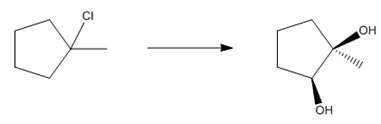# Problem: Show how you would carry out each of the following reactions. You do   NOT need to draw the mechanisms

###### FREE Expert Solution
89% (500 ratings)
###### Problem Details

Show how you would carry out each of the following reactions. You do   NOT need to draw the mechanismsFrequently Asked Questions

What scientific concept do you need to know in order to solve this problem?

Our tutors have indicated that to solve this problem you will need to apply the Retrosynthesis concept. You can view video lessons to learn Retrosynthesis. Or if you need more Retrosynthesis practice, you can also practice Retrosynthesis practice problems.

What is the difficulty of this problem?

Our tutors rated the difficulty ofShow how you would carry out each of the following reactions...as medium difficulty.

How long does this problem take to solve?

Our expert Organic tutor, Jonathan took 2 minutes and 15 seconds to solve this problem. You can follow their steps in the video explanation above.